# ‘fourierin’ package

#### 2019-04-04

This is a package in R to numerically calculate Fourier-type integrals of multivariate functions with compact support evaluated at regular grids. Specifically, integrals of the type

$I \left[f(t), \boldsymbol{a}, \boldsymbol{b};r, s \right] = \left[ \frac{|s|}{(2\pi)^{1 - r}}\right]^{n/2} \int_{a_1}^{b_1}\int_{a_2}^{b_2}\cdots\int_{a_n}^{b_n} f(\boldsymbol{t})e^{\imath s \langle \boldsymbol{w}, \boldsymbol{t}\rangle} \text{d}\boldsymbol{t},$

where,

$$\boldsymbol{a} = (a_1, \ldots, a_n)$$, $$\boldsymbol{b} = (b_1, \ldots, b_n)$$, $$\boldsymbol{t} = (t^{(1)}, \ldots, t^{(n)})$$, $$\boldsymbol{w} = (w^{(1)}, \ldots, w^{(n)})$$, $$a_l \leq t^{(l)} \leq b_l$$, $$c_l \leq w^{(l)} \leq c_l$$.

Common values for r are -1, 0 and -1, while common values for s are -2, -1, 1 and 2. For example, if f is a density function, s = 1 and r = 1 could be used to obtain the characteristic function of f. Conversely, if f is the characteristic function of a probability density function, then r = -1 and s = -1 could be used to recover the density.

The implementation of this algorithm is the one described in Inverarity (2002), Fast Computation of multidimensional Integrals.

Some examples (also found in documentation).

## Example 0: Speed tests

Below it is an example of univariate continuous Fourier transform.

library(fourierin)
library(dplyr)
##
## Attaching package: 'dplyr'
## The following objects are masked from 'package:stats':
##
##     filter, lag
## The following objects are masked from 'package:base':
##
##     intersect, setdiff, setequal, union
library(purrr)
library(ggplot2)

## Test speed at several resolutions
resolution <- 2^(3:8)

## Function to be tested
myfnc <- function(t) exp(-t^2/2)

## Aux. function
compute_times <- function(resol){
reps <- 100
rbenchmark::benchmark(
yes = fourierin_1d(f = myfnc, -5, 5, -3, 3, -1, -1, resol),
no = fourierin_1d(f = myfnc, -5, 5, -3, 3, -1, -1, resol,
use_fft = FALSE),
replications = reps) %>%
as.data.frame() %>%
select(FFT = test, time = elapsed) %>%
mutate(time = time*1e3/reps, resolution = resol)
}

resolution %>%
map_df(compute_times) %>%
mutate(resolution = as.factor(resolution)) %>%
ggplot(aes(resolution, time, color = FFT)) +
geom_point(size = 2, aes(shape = FFT)) +
geom_line(aes(linetype = FFT, group = FFT)) +
ylab("time (in milliseconds)")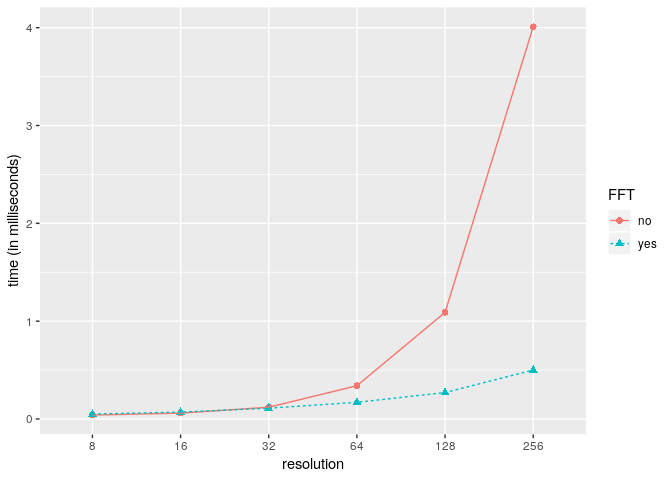Now we present an example using a bivariate function.

## Load packages
library(fourierin)
library(dplyr)
library(purrr)
library(ggplot2)

## Test speed at several resolutions
resolution <- 2^(3:7)

## Bivariate function to be tested
myfnc <- function(x) dnorm(x[, 1])*dnorm(x[, 2])

## Aux. function
compute_times <- function(resol){
reps <- 1
resol <- rep(resol, 2)
rbenchmark::benchmark(
yes = fourierin(myfnc,
lower_int = c(-8, -6), upper_int = c(6, 8),
lower_eval = c(-4, -4), upper_eval = c(4, 4),
resolution = resol),
no = fourierin(myfnc,
lower_int = c(-8, -6), upper_int = c(6, 8),
lower_eval = c(-4, -4), upper_eval = c(4, 4),
resolution = resol, use_fft = FALSE),
replications = reps) %>%
as.data.frame() %>%
select(FFT = test, time = elapsed) %>%
mutate(time = time/reps, resolution = resol)
}

## Values
comparison <-
resolution %>%
map_df(compute_times)

fctr_order <-
unique(comparison$resolution) %>% paste(., ., sep = "x") ## Plot comparison %>% mutate(resolution = paste(resolution, resolution, sep = "x"), resolution = ordered(resolution, levels = fctr_order)) %>% ggplot(aes(resolution, time, color = FFT)) + geom_point(size = 2, aes(shape = FFT)) + geom_line(aes(linetype = FFT, group = FFT)) + ylab("time (in seconds)")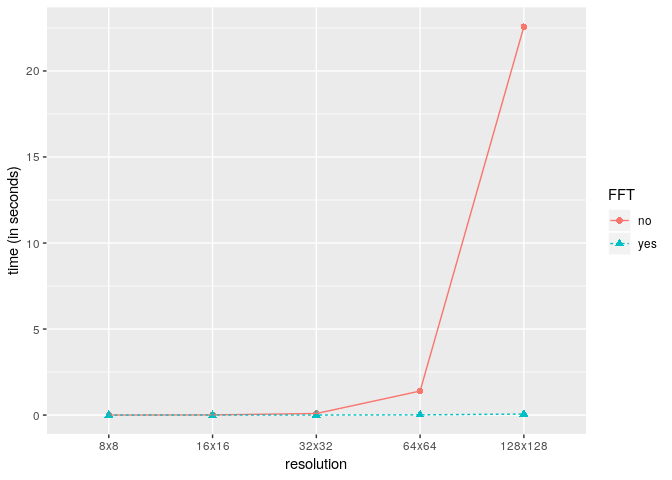## Example 1: Recovering standard normal from its characteristic function The density function of a random variable can be recovered using the Fourier inversion theorem. The characteristic function of a standard normal distribution is $\phi(t) = \exp(-t^2/2) \;\; t \in \mathbb{R}$ The due to the smoothness and symmetry of both, the characteristic function and the standard normal density, it can be well approximated even with a low resolution. library(fourierin) library(dplyr) library(ggplot2) ## Characteristic function of std. normal. fnc <- function(t) exp(-t^2/2) ## Compute integral out <- fourierin(f = fnc, lower_int = -5, upper_int = 5, lower_eval = -5, upper_eval = 5, const_adj = -1, freq_adj = -1, resolution = 64) ## Extract values and compute true values of the density df1 <- out %>% as_data_frame() %>% mutate(values = Re(values), density = "approximated") ## Warning: as_data_frame() is deprecated, use as_tibble() (but mind the new semantics). ## This warning is displayed once per session. df2 <- data_frame(w = seq(min(df1$w), max(df1$w), len = 150), values = dnorm(w), density = "true") ## Warning: data_frame() is deprecated, use tibble(). ## This warning is displayed once per session. bind_rows(df1, df2) %>% ggplot(aes(w, values, color = density)) + geom_line(aes(linetype = density)) + xlab("x") + ylab("f(x)")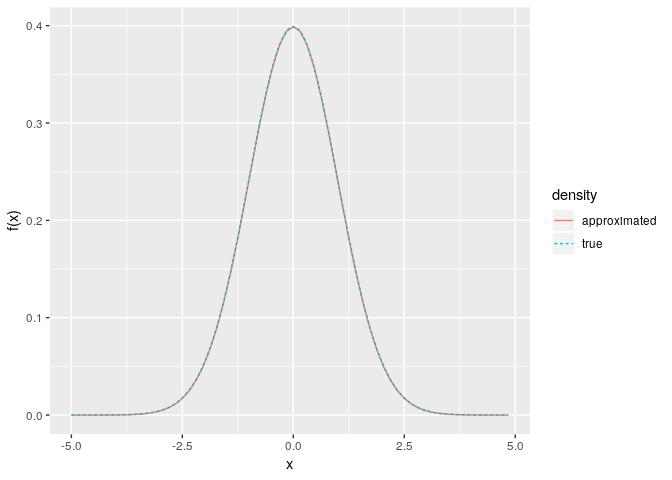We now present a second example: recovering the density of a $$\chi^2$$ distribution using its characteristic function. The corresponding density is not symmetric and its support is not the entire real line, thus a higher resolution might be required to achieve a good approximation. library(fourierin) library(dplyr) library(purrr) library(ggplot2) ## Set functions df <- 5 cf <- function(t) (1 - 2i*t)^(-df/2) dens <- function(x) dchisq(x, df) ## Set resolutions resolutions <- 2^(6:8) ## Compute integral given the resoltion recover_f <- function(resol){ ## Get grid and density values out <- fourierin(f = cf, lower_int = -10, upper_int = 10, lower_eval = -3, upper_eval = 20, const_adj = -1, freq_adj = -1, resolution = resol) ## Return in dataframe format out %>% as_data_frame() %>% transmute( x = w, values = Re(values), resolution = resol) } ## Density approximations vals <- map_df(resolutions, recover_f) ## True values true <- data_frame(x = seq(min(vals$x), max(vals$x), length = 150), values = dens(x)) vals %>% mutate(resolution = ordered(resolution, labels = resolutions)) %>% ggplot(aes(x, values)) + geom_line(aes(color = resolution)) + geom_line(data = true, aes(color = "true values"))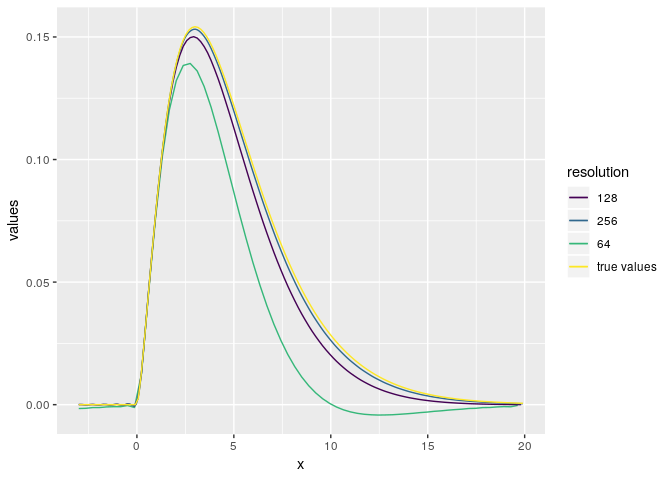## Example 2: Computing characteristic function of a gamma density library(fourierin) library(tidyr) library(dplyr) library(purrr) library(ggplot2) ## Compute integral shape <- 5 rate <- 3 myfnc <- function(t) dgamma(t, shape, rate) ## Function to compute characteristic function for a given resolution. approximate_CF <- function (resol) { out <- fourierin(f = myfnc, -0, 8, -5, 5, 1, 1, resol) out %>% as_data_frame() %>% transmute(t = w, real = Re(values), imaginary = Im(values), resolution = as.character(resol)) %>% gather(CF, values, -t, -resolution) } ## Evaluate approxs. to CF for different resulutions resolution <- 2^c(6, 7) CF <- map_df(resolution, approximate_CF) ## Evaluate true values of characteristic function true_cf <- function(t, shape, rate) (1 - 1i*t/rate)^-shape true <- data_frame(t = seq(min(CF$t), max(CF\$t), length = 150),
values = true_cf(t, shape, rate),
real = Re(values),
imaginary = Im(values),
resolution = "true values") %>%
select(-values) %>%
gather(CF, values, -t, -resolution)

## Plot values
CF %>%
ggplot(aes(t, values, color = resolution,
group = resolution)) +
geom_line(aes()) +
geom_line(data = true) +
facet_grid(~CF) +
theme(legend.position = "bottom")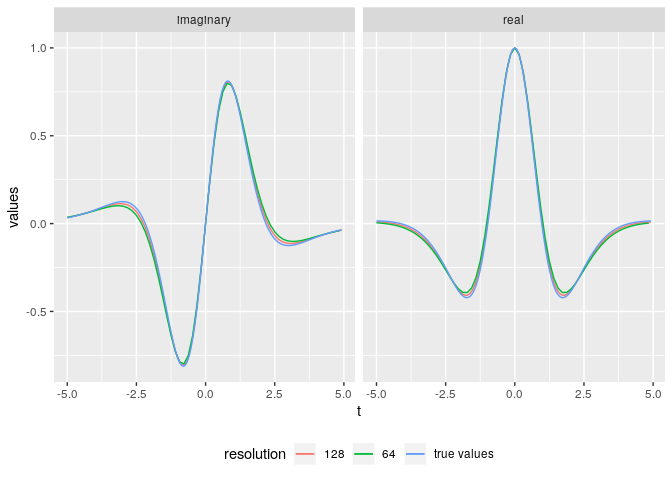## Example 3: Recovering std. normal from its characteristic function

Testing example.

## Load packages
library(fourierin)
library(tidyr)
library(dplyr)
library(purrr)
library(lattice)
library(ggplot2)

## Set functions to be tested with their corresponding parameters.
mu <- c(-1, 1)
sig <- matrix(c(3, -1, -1, 2), 2, 2)

## Multivariate normal density, x is n x d
f <- function(x) {
## Auxiliar values
d <- ncol(x)
z <- sweep(x, 2, mu, "-")
## Get numerator and denominator of normal density
num <- exp(-0.5*rowSums(z * (z %*% solve(sig))))
denom <- sqrt((2*pi)^d*det(sig))
return(num/denom)
}

## Characteristic function, s is n x d
phi <- function (s) {
complex(modulus = exp(-0.5*rowSums(s*(s %*% sig))),
argument = s %*% mu)
}

## Evaluate characteristic function for a given resolution.
eval <- fourierin(f,
lower_int = c(-8, -6), upper_int = c(6, 8),
lower_eval = c(-4, -4), upper_eval = c(4, 4),
use_fft = T)

## --- Little test
dat <- eval %>%
with(crossing(y = w2, x = w1) %>%
mutate(approximated = c(values))) %>%
mutate(true = phi(matrix(c(x, y), ncol = 2)),
difference = approximated - true) %>%
gather(value, z, -x, -y) %>%
mutate(real = Re(z), imaginary = Im(z)) %>%
select(-z) %>%
gather(part, z, -x, -y, -value)

## Surface plot
wireframe(z ~ x*y | value*part, data = dat,
scales =
list(arrows=FALSE, cex= 0.45,
col = "black", font = 3, tck = 1),
screen = list(z = 90, x = -74),
colorkey = FALSE,
light.source= c(0,10,10),
height, w = 0.4)
grey(w*irr + (1 - w)*(1 - (1 - ref)^0.4)),
aspect = c(1, 0.65))

## Contours of values
dat %>%
filter(value != "difference") %>%
ggplot(aes(x, y, z = z)) +
geom_tile(aes(fill = z)) +
facet_grid(part ~ value) +
scale_fill_distiller(palette = "Reds")

## Contour of differences
dat %>%
filter(value == "difference") %>%
ggplot(aes(x, y, z = z)) +
geom_tile(aes(fill = z)) +
facet_grid(part ~ value) +
scale_fill_distiller(palette = "Spectral")